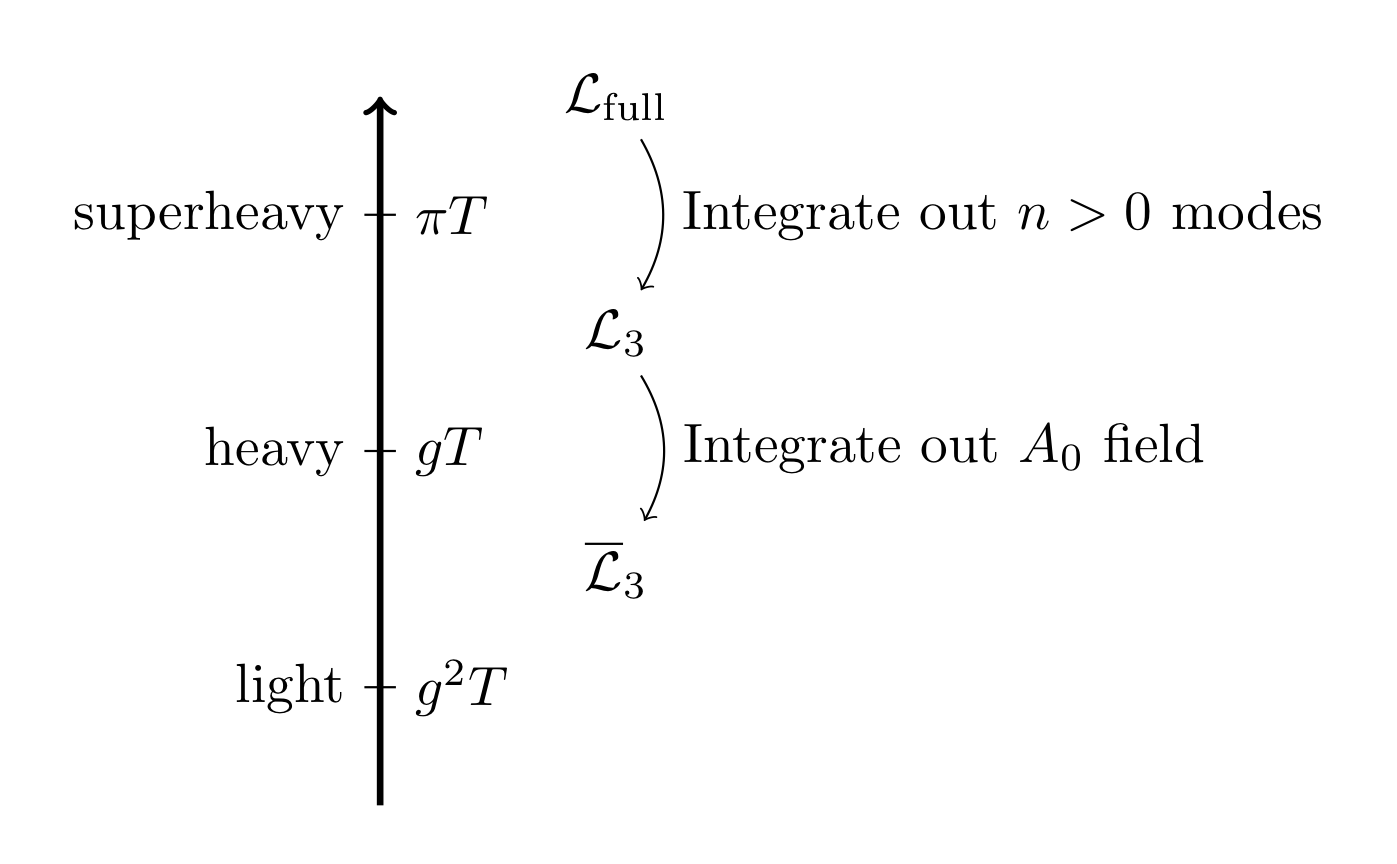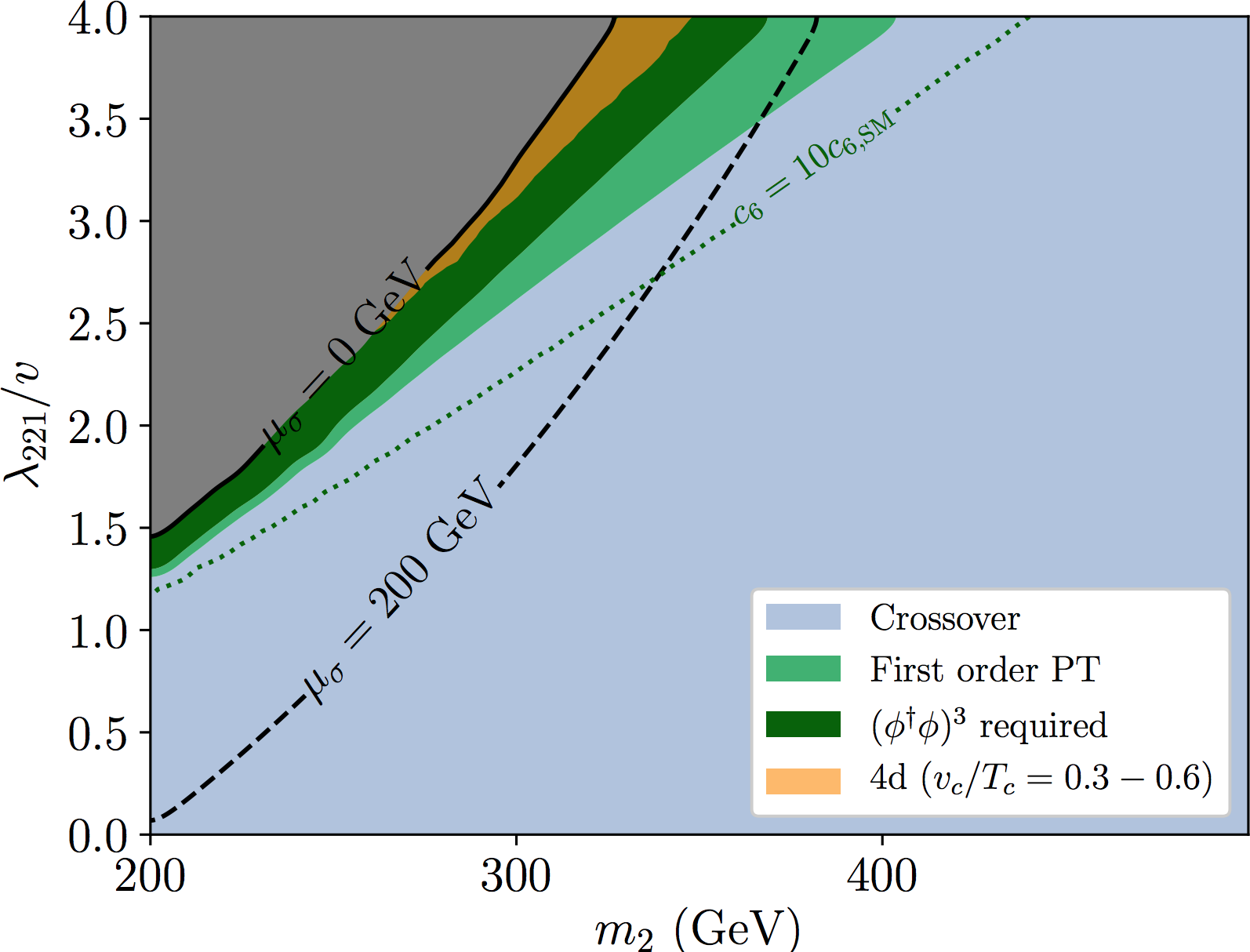### End-to-end studies of the electroweak phase transition

David Weir [he/him] - davidjamesweir

University of Helsinki

saoghal.net/slides/kcl

King's College London, 4 December 2019

#### Start of the GW astrophysics era

Source:
(CC-BY) arXiv:1710.05833

### After LIGO comes LISA

• Three laser arms, 2.5 M km separation
• ESA-NASA mission, launch by 2034

### LISA: Early Universe Cosmology

Science Investigation 7.2: Measure, or set upper limits on, the spectral shape of the cosmological stochastic GW background.

Operational Requirement 7.2: Probe a broken power-law stochastic background from the early Universe as predicted, for example, by first order phase transitions ...

### Electroweak phase transition

• Process by which the Higgs 'switched on'
• In the Standard Model it is a crossover
• Possible in extensions that it would be first order
➥ colliding bubbles then make gravitational waves
• Can also be probed with experimental particle physics

Source: arXiv:1206.2942

### EW PT in the SM

Work in the 1990s found this phase diagram for the SM:

At $m_H = 125 \, \mathrm{GeV}$, SM is a crossover
Kajantie et al.; Gurtler et al.; Csikor et al.; ...

### How? Dimensional reduction

• At high $T$, system looks 3D at distances $\Delta x \gg 1/T$
• Decomposition of fields into Matsubara modes: $$\phi(\tau, \mathbf{x}) = T \sum_{n=-\infty}^{\infty} \tilde{\phi}_n(\mathbf{x}) e^{i \omega_n \tau}; \quad \omega_n = \begin{cases} 2n \pi T & \text{bosons} \\ (2n +1) \pi & \text{fermions} \end{cases}$$
• Integrate out $\omega_n\neq 0$ due to scale separation
(heavy modes $\gtrsim \pi T$) $$Z = \int \mathcal{D} \phi_0 \mathcal{D} \phi_n e^{-S(\phi_0) - S(\phi_0, \phi_n)} \to \int \mathcal{D} \phi_0 e^{-S(\phi_0) - S_\text{eff}(\phi_0)}$$
• Also handles the infrared problem, leaving light fields to be studied nonperturbatively.

### Dimensional reduction: sketchEach step involves matching Green's functions in the effective and full theories to the desired order.

### Using the dimensional reduction

• Simulate DR'ed 3D theory on lattice Kajantie et al.
• Applied to SM very successfully in the 1990s:
• NB: if 'new physics' heavy, can be integrated out in DR

### DR: xSM example

[$Z_2$-symmetric; plot of trilinear higgs-singlet coupling $\lambda_{221}$ vs. singlet mass eigenstate $m_2$]Source: arXiv:1903.11604 [with Oliver Gould, Jonathan Kozaczuk,
Lauri Niemi, Michael Ramsey-Musolf and Tuomas Tenkanen]

### Out of equilibrium physics

1. Bubbles nucleate and grow
2. Expand in a plasma - create reaction fronts
3. Bubbles + fronts collide - violent process
4. Sound waves left behind in plasma
5. Turbulence; damping

### How the wall moves

• In EWPT: equation of motion is (schematically)
$$\partial^2 \phi + V_\text{eff}'(\phi,T) + \sum_{i} \frac{d m_i^2}{d \phi} \int \frac{\mathrm{d}^3 k}{(2\pi)^3 \, 2 E_i} \delta f_i(\mathbf{k},\mathbf{x}) = 0$$
• $V_\text{eff}'(\phi)$: gradient of finite-$T$ effective potential
• $\delta f_i(\mathbf{k},\mathbf{x})$: deviation from equilibrium phase space density of $i$th species
• $m_i$: effective mass of $i$th species

### Force interpretation

$$\overbrace{\partial_\mu T^{\mu\nu}}^\text{Force on \phi} - \overbrace{\int \frac{d^3 k}{(2\pi)^3} f(\mathbf{k}) F^\nu }^\text{Force on particles}= 0$$

This equation is the realisation of this idea:

### Field-fluid system

Using a flow ansatz for the wall-plasma system:

$$\overbrace{\partial_\mu T^{\mu\nu}}^\text{Field part} - \overbrace{\int \frac{d^3 k}{(2\pi)^3} f(\mathbf{k}) F^\nu }^\text{Fluid part}= 0$$

i.e.:

$$\partial_\mu T^{\mu\nu}_\phi + \partial_\mu T^{\mu\nu}_\text{fluid} = 0$$

Can simulate as effective model of field $\phi$ + fluid $u^\mu$

astro-ph/9309059

### Key parameters for GW production

• $T_*$, temperature
• $T_* \sim 100 \, \mathrm{GeV} \longrightarrow \mathrm{mHz}$ today
• $\alpha_{T_*}$, vacuum energy fraction
• $\alpha_{T_*} \ll 1$: 'weak'
• $\alpha_{T_*} \gtrsim 1$: 'strong'
• $v_\mathrm{w}$, bubble wall speed
• $\beta/H_*$, 'duration'
• $\beta$: inverse phase transition duration
• $H_*$: Hubble rate at transition

### What sources GWs?

• Bubbles nucleate + expand, reaction fronts form, then:
1. $h^2 \Omega_\phi$: Bubbles + shocks collide - 'envelope phase'
2. $h^2 \Omega_\text{sw}$: Sound waves set up - 'acoustic phase'
3. $h^2 \Omega_\text{turb}$: [MHD] turbulence - 'turbulent phase'

• Sources add together to give observed GW power: $$h^2 \Omega_\text{GW} + h^2 \Omega_\text{sw} + h^2 \Omega_\text{turb}$$
• Bubbles nucleate + expand, reaction fronts form, then:
1. $h^2 \Omega_\phi$: Bubbles + shocks collide - 'envelope phase'
2. $h^2 \Omega_\text{sw}$: Sound waves set up - 'acoustic phase'
3. $h^2 \Omega_\text{turb}$: [MHD] turbulence - 'turbulent phase'

• Sources add together to give observed GW power: $$h^2 \Omega_\text{GW} + h^2 \Omega_\text{sw} + h^2 \Omega_\text{turb}$$

### Why focus on sound waves?

• "Envelope phase" is short-lived, one-off
• Relevant only in exotic models where $\alpha_{T_*} \gg 1$ or vacuum transitions...
• ... but then dynamics aren't so simple arXiv:1802.05712
• "Turbulent phase" may never occur if Hubble damping happens on a shorter timescale
• Turbulence: bubble radius/average fluid velocity
• Hubble damping: Hubble time

➤ Focus on the hydrodynamics of the transition
and immediate aftermath.

## Weak transitions

### $\alpha_{T_*} \ll 1$

[with Mark Hindmarsh, Stephan Huber,
Kari Rummukainen + LISA CosWG]

### Velocity profile development: detonation vs deflagration

Deflagration $v_\mathrm{w} < c_\mathrm{s}$

### Simulation slice example

[$\alpha_{T_*} = 0.01$, $v_\mathrm{w} = 0.68$ (detonation)]

### Velocity power spectra

$v_\mathrm{w} < c_\mathrm{s}$

### GW power spectra

[curves scaled by $t$]

$v_\mathrm{w} < c_\mathrm{s}$

### Putting it all together - $h^2 \Omega_\text{gw}$

• For any theory, can get $T_*$, $\alpha_{T_*}$, $\beta/H_*$, $v_\mathrm{w}$ arXiv:1004.4187
• It's then easy to predict the signal...

(example, $T_* = 200~\mathrm{GeV}$, $\alpha_{T_*} = 0.1$, $v_\mathrm{w} =0.9$, $\beta/H_* = 50$) $\mathrm{SNR} = 27$ ☺️
[From ptplot.org / arXiv:1910.13125]

### PTPlot.org

Model ⟶ ($T_*$, $\alpha_{T_*}$, $v_\mathrm{w}$, $\beta$) ⟶ this plot

[Here: $Z_2$-symmetric xSM points from arXiv:1910.13125]

### GW reach of SM EFT

Source: arXiv:1903.11604

## Strong transitions

### $\alpha_{T_*} \sim 1$

arXiv:1906.00480

[with Daniel Cutting, Mark Hindmarsh]

### Fluid profile around the wall

Deflagration $v_\mathrm{w} < c_\mathrm{s}$

NB: Deflagration front ends at a shock ($\approx c_\mathrm{s}$)

[Movies: Cosmic Defects Channel]

Deflagration $v_\mathrm{w} < c_\mathrm{s}$

NB: Deflagration front ends at a shock ($\approx c_\mathrm{s}$)

[Movies: Cosmic Defects Channel]

### Strong simulation slice 1

[$\alpha_{T_*} = 0.5$, $v_\mathrm{w} = 0.92$ (detonation)], velocity $\mathbf{v}$

### Fluid profile around the wall

Deflagration $v_\mathrm{w} < c_\mathrm{s}$

NB: Deflagration front ends at a shock ($\approx c_\mathrm{s}$)

[Movies: Cosmic Defects Channel]

### Strong simulation slice 2

[$\alpha_{T_*} = 0.5$, $v_\mathrm{w} = 0.44$ (deflag.)], velocity $\mathbf{v}$

### Strong deflagrations: walls slow

At large $\alpha_{T_*}$ reheated droplets form in front of the walls

### Strong simulation slice 3

[$\alpha_{T_*} = 0.34$, $v_\mathrm{w} = 0.24$ (deflag.)], vorticity $\nabla \times \mathbf{v}$

### GW production can be suppressed

Suppression relative to LISA CosWG ansatz

### 🚰 A pipeline

1. Choose your model (e.g. SM, xSM, 2HDM, ...)
2. Dim. red. model
Kajantie et al.
3. Phase diagram ($\alpha_{T_*}$, $T_*$);
lattice: Kajantie et al.
4. Nucleation rate ($\beta$) + Sphaleron rate;
lattice: Moore and Rummukainen
5. Wall velocities ($v_\text{wall}$)
Moore and Prokopec; Kozaczuk
6. GW power spectrum $\Omega_\mathrm{gw}$

🔧 Not watertight, even for SM!

### Conclusions

• Weak transitions: good estimates of power spectrum
ptplot.org
• Strong transitions still an emerging topic:
☛ acoustic gravitational wave production suppressed
• Causes: vortical mode production and slower walls
• Worst for large $\alpha_{T_*}$, small $v_\mathrm{w}$
• Turbulence still a challenge, work ongoing
• In any case: LISA provides a model-independent probe of first-order phase transitions around $100~\mathrm{GeV}$
☛ ... but new physics must be dynamical